## Ford Circle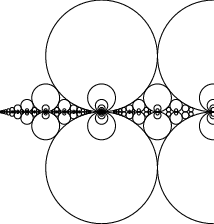Pick any two Integersand, then the Circle of Radiuscentered atis known as a Ford circle. No matter what and how manys ands are picked, none of the Ford circles intersect (and all are tangent to the x-Axis). This can be seen by examining the squared distance between the centers of the circles with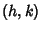and,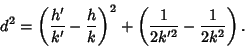(1)

Letbe the sum of the radii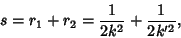(2)

then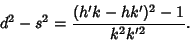(3)

But, soand the distance between circle centers isthe sum of the Circle Radii, with equality (and therefore tangency) Iff. Ford circles are related to the Farey Sequence (Conway and Guy 1996).

References

Conway, J. H. and Guy, R. K. Farey Fractions and Ford Circles.'' The Book of Numbers. New York: Springer-Verlag, pp. 152-154, 1996.

Ford, L. R. Fractions.'' Amer. Math. Monthly 45, 586-601, 1938.

Pickover, C. A. Fractal Milkshakes and Infinite Archery.'' Ch. 14 in Keys to Infinity. New York: W. H. Freeman, pp. 117-125, 1995.

Rademacher, H. Higher Mathematics from an Elementary Point of View. Boston, MA: Birkhäuser, 1983.